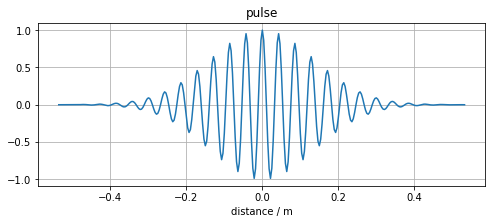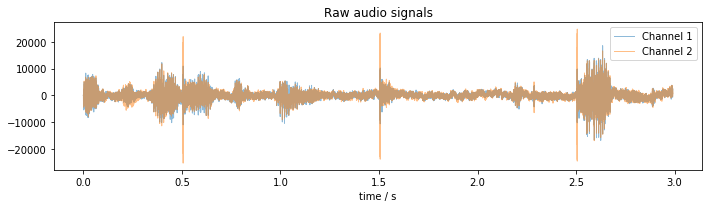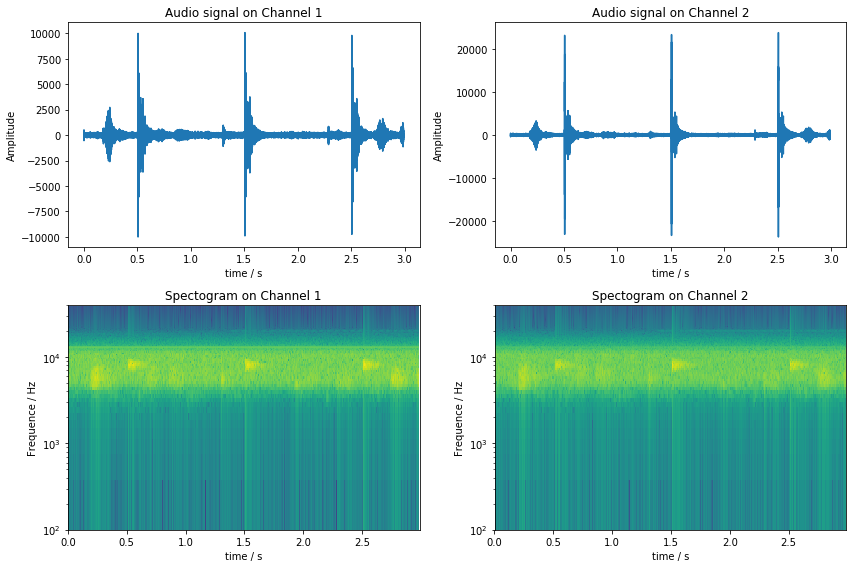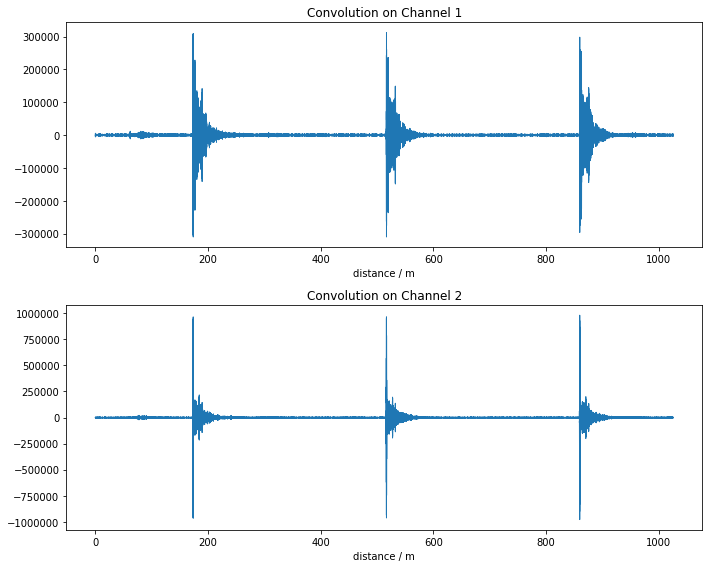Echo analysis of a high-pitch pulse¶

In :
import matplotlib.pyplot as plt
import numpy as np
from scipy import signal

# Uncomment to plot into an interactive window
# %matplotlib qt5

import echorec
exe = '../build/src/echorec'
In :
speedofsound = 343.0 # m/s
samplerate = 96000 # samples per second
m_per_sample = speedofsound / samplerate

The pulse shape¶

We send out a pulse a couple of times. The pulse is a cosine with a Gaussian window.

In :
# Base frequency of the pulse's cosine
pulsefreq = samplerate / 12.0
# The sigma of the Gaussian window
pulsesigma = 0.0004

@np.vectorize
def pulse(t):
"""The pulse signal as a function of time."""
ampl = np.exp(- t**2 / (2.0 * pulsesigma**2));
return ampl * np.cos(t * pulsefreq * 2.0 * np.pi);

fig, ax = plt.subplots(figsize=(8, 3))
ts = np.arange(-150, 150) / samplerate
ax.plot(speedofsound * ts, pulse(ts))
ax.set_title("pulse")
ax.set_xlabel("distance / m")
ax.grid()
plt.show()Capturing the echo¶

We invoke the pulseaudio-based tool echorec to send out the pulse a given number of times and simultaneously record the acoustic feedback.

You can launch pavucontorl and switch to "Input Devices" in parallel to have the recording start immediately.

In :
res = echorec.echorec_lambda(pulse, -0.5, 0.5, samplerate, count=3, exe=exe)
rawdata = np.frombuffer(res, dtype=np.int16)

# rawdata = np.fromfile("/tmp/rec.dat", dtype=np.int16)

channels = [rawdata[0::2], rawdata[1::2]]
sample_count = len(channels)
In :
ts = np.arange(sample_count) / samplerate
ps = pulse(np.arange(-100, 100) / samplerate)

fig, ax = plt.subplots(figsize=(10, 3))
for chidx in range(2):
ch = channels[chidx]
chname = "Channel {}".format(chidx + 1)
ax.plot(ts, ch, label=chname, lw=1, alpha=0.5)
ax.set_xlabel("time / s")
ax.set_title("Raw audio signals")
ax.legend()

fig.tight_layout()
plt.show()Signal processing¶

Bandpass filter¶

In :
# Band pass filtering
if True:
nyq = (0.5 * samplerate)
lo, hi = np.array([pulsefreq-3000.0, pulsefreq+3000.0]) / nyq
b, a = signal.butter(8, [lo, hi], btype='band', analog=False)

for i in range(2):
channels[i] = signal.lfilter(b, a, channels[i])
In :
fig, axes = plt.subplots(2, 2, figsize=(12, 8))

for chidx in range(2):
ch = channels[chidx]
chname = "Channel {}".format(chidx + 1)

ax = axes[chidx]
ax.plot(ts, ch, label='ch1')
ax.set_xlabel("time / s")
ax.set_ylabel("Amplitude")
ax.set_title("Audio signal on {}".format(chname))

ax = axes[chidx]
ax.specgram(ch, Fs=samplerate)
ax.set_yscale('log')
ax.set_ylim((100, 40000))

ax.set_xlabel("time / s")
ax.set_ylabel("Frequence / Hz")
ax.set_title("Spectogram on {}".format(chname))

fig.tight_layout()
plt.show()Convolution with pulse signal¶

In :
fig, axes = plt.subplots(2, figsize=(10, 8))

for chidx in range(2):
ch = channels[chidx]
chname = "Channel {}".format(chidx + 1)

ax = axes[chidx]
ax.plot(speedofsound * ts, np.convolve(ch, ps, mode='same'), label='conv', lw=1)
ax.set_xlabel("distance / m")
ax.set_title("Convolution on {}".format(chname))

fig.tight_layout()
plt.show()